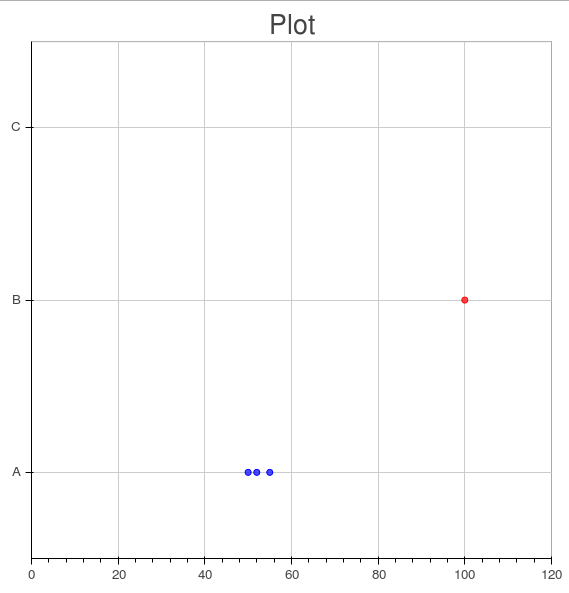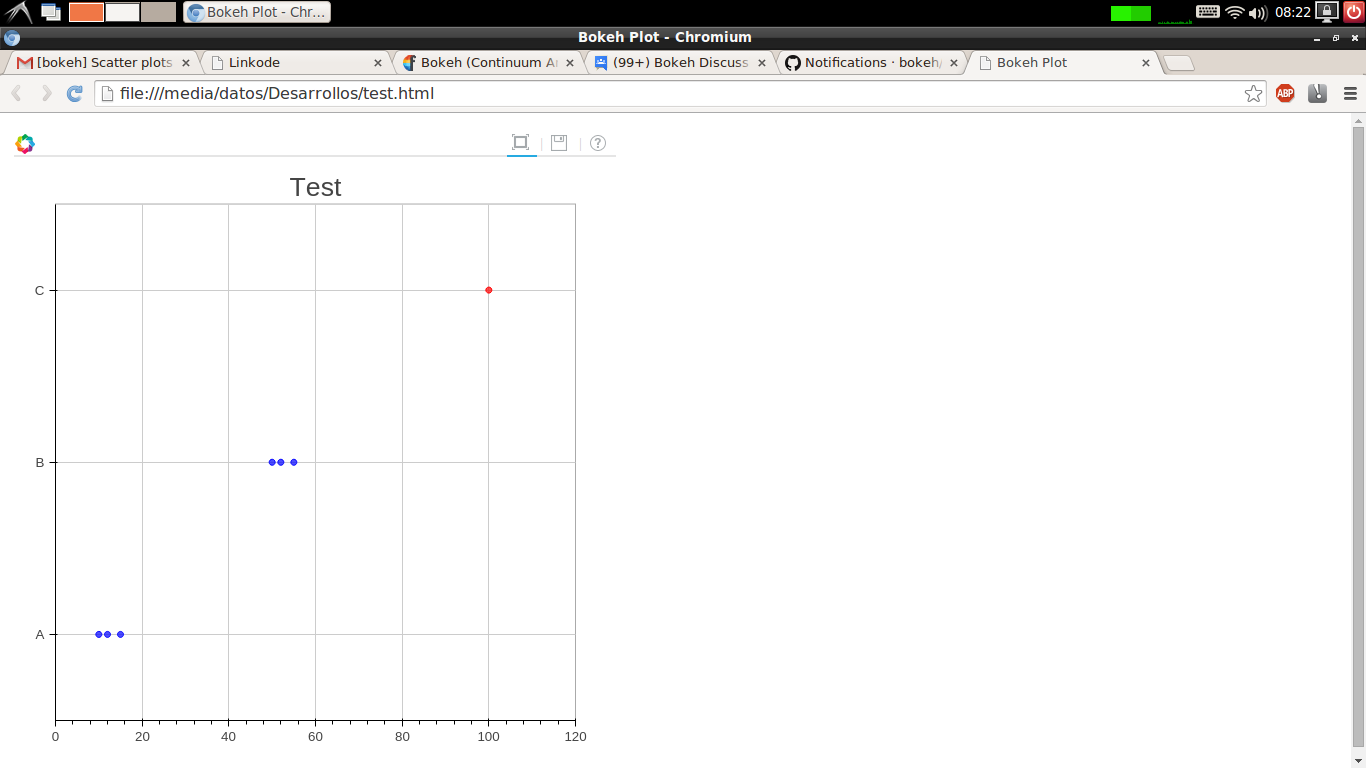# Scatter plots with multiple points

Hi!

I'm trying to graph some prices according to the location where the
prices are taken.

This is the code :

"""
from bokeh.plotting import *

output_file("test.html")

# the data, very simple, a location name and a price
data = [
("A", 10),
("B", 50),
("C", 100),
("A", 12),
("A", 15),
("B", 55),
("B", 52),
]
# a list of all locations, ordered
locations = sorted({x for x in data})

x =
y =
c =
# for each data point, add to three lists: the price, the location (but using a
# number, otherwise scatter doesn't work), and the color according to the price
for locat, price in data:
x.append(price)
y.append(locations.index(locat))
c.append("red" if price > 70 else 'blue')

# plot
scatter(x, y, color=c, fill_alpha=0.7, size=6, y_range=locations,
x_range=[0, 120])
show()
"""

It creates the attached image.

As you can see, there are two problems with it:

1. there is one row without points!

2. the row name doesn't correspond with the data.

Probably I'm using badly the scatter plot, but I couldn't find the problem.

Help? Thanks!Regards,···

--
. Facundo

Blog: http://www.taniquetil.com.ar/plog/
PyAr: http://www.python.org/ar/

Hey Facu,

Nice to see you here…

I am pasting the solution below but I am also posting a linkode  to see it better.···

On Fri, Dec 12, 2014 at 7:46 PM, Facundo Batista [email protected] wrote:

Hi!

I’m trying to graph some prices according to the location where the

prices are taken.

This is the code :

“”"

from bokeh.plotting import *

output_file(“test.html”)

# the data, very simple, a location name and a price

data = [

``````("A", 10),

("B", 50),

("C", 100),

("A", 12),

("A", 15),

("B", 55),

("B", 52),
``````

]

# a list of all locations, ordered

locations = sorted({x for x in data})

x =

y =

c =

# number, otherwise scatter doesn’t work), and the color according to the price

for locat, price in data:

``````x.append(price)

y.append(locations.index(locat))

c.append("red" if price > 70 else 'blue')
``````

# plot

scatter(x, y, color=c, fill_alpha=0.7, size=6, y_range=locations,

x_range=[0, 120])

show()

“”"

It creates the attached image.

As you can see, there are two problems with it:

1. there is one row without points!

2. the row name doesn’t correspond with the data.

Probably I’m using badly the scatter plot, but I couldn’t find the problem.

Help? Thanks!Regards,

. Facundo

You received this message because you are subscribed to the Google Groups “Bokeh Discussion - Public” group.

To unsubscribe from this group and stop receiving emails from it, send an email to [email protected].

To post to this group, send email to [email protected].

To view this discussion on the web visit https://groups.google.com/a/continuum.io/d/msgid/bokeh/CAM09pzRM6nxH-V7%2BfG6zxbFZySnGFLZmiC0Lpwc9YWgH5BxV%2BA%40mail.gmail.com.

I am pasting the solution below but I am also posting a linkode  to see
it better.

It works!! Thanks1) you are using the new plotting API, which is better than the previous one
(now you create an figure object and add elements to it)
2) all the sources have the same lengths (x, locations, colors)
3) pass the a unique locations list to y_range to restrict the actual
categories to ["A", "B", "C"]

Btw, I deliberated change the name of some variables to help you understand
better.

Awesome, thank you very much!

Regards,

···

On Sat, Dec 13, 2014 at 8:25 AM, Damian Avila <[email protected]> wrote:

--
. Facundo

Blog: http://www.taniquetil.com.ar/plog/
PyAr: http://www.python.org/ar/